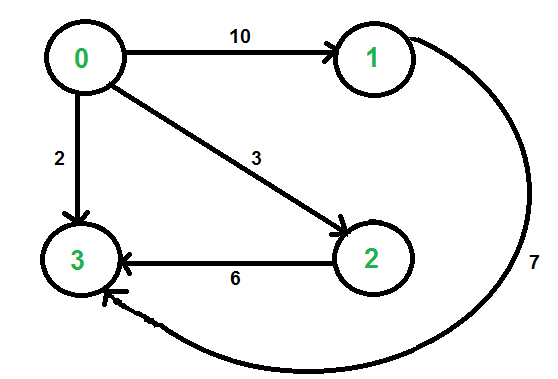# Graph implementation using STL for competitive programming | Set 2 (Weighted graph)

In Set 1, unweighted graph is discussed. In this post, weighted graph representation using STL is discussed. The implementation is for adjacency list representation of weighted graph.We use two STL containers to represent graph:

• vector : A sequence container. Here we use it to store adjacency lists of all vertices. We use vertex number as index in this vector.
• pair : A simple container to store pair of elements. Here we use it to store adjacent vertex number and weight of edge connecting to the adjacent.

The idea is to use a vector of pair vectors. Below code implements the same.

 `// C++ program to represent undirected and weighted graph ` `// using STL. The program basically prints adjacency list ` `// representation of graph ` `#include ` `using` `namespace` `std; ` ` `  `// To add an edge ` `void` `addEdge(vector > adj[], ``int` `u, ` `                                     ``int` `v, ``int` `wt) ` `{ ` `    ``adj[u].push_back(make_pair(v, wt)); ` `    ``adj[v].push_back(make_pair(u, wt)); ` `} ` ` `  `// Print adjacency list representaion ot graph ` `void` `printGraph(vector > adj[], ``int` `V) ` `{ ` `    ``int` `v, w; ` `    ``for` `(``int` `u = 0; u < V; u++) ` `    ``{ ` `        ``cout << ``"Node "` `<< u << ``" makes an edge with \n"``; ` `        ``for` `(``auto` `it = adj[u].begin(); it!=adj[u].end(); it++) ` `        ``{ ` `            ``v = it->first; ` `            ``w = it->second; ` `            ``cout << ``"\tNode "` `<< v << ``" with edge weight ="` `                 ``<< w << ``"\n"``; ` `        ``} ` `        ``cout << ``"\n"``; ` `    ``} ` `} ` ` `  `// Driver code ` `int` `main() ` `{ ` `    ``int` `V = 5; ` `    ``vector > adj[V]; ` `    ``addEdge(adj, 0, 1, 10); ` `    ``addEdge(adj, 0, 4, 20); ` `    ``addEdge(adj, 1, 2, 30); ` `    ``addEdge(adj, 1, 3, 40); ` `    ``addEdge(adj, 1, 4, 50); ` `    ``addEdge(adj, 2, 3, 60); ` `    ``addEdge(adj, 3, 4, 70); ` `    ``printGraph(adj, V); ` `    ``return` `0; ` `} `

Output:

```Node 0 makes an edge with
Node 1 with edge weight =10
Node 4 with edge weight =20

Node 1 makes an edge with
Node 0 with edge weight =10
Node 2 with edge weight =30
Node 3 with edge weight =40
Node 4 with edge weight =50

Node 2 makes an edge with
Node 1 with edge weight =30
Node 3 with edge weight =60

Node 3 makes an edge with
Node 1 with edge weight =40
Node 2 with edge weight =60
Node 4 with edge weight =70

Node 4 makes an edge with
Node 0 with edge weight =20
Node 1 with edge weight =50
Node 3 with edge weight =70

```

This article is contributed by Sahil Chhabra (akku). If you like GeeksforGeeks and would like to contribute, you can also write an article using contribute.geeksforgeeks.org or mail your article to contribute@geeksforgeeks.org. See your article appearing on the GeeksforGeeks main page and help other Geeks.My Personal Notes arrow_drop_up

Improved By : Ritesh Ghorse

Article Tags :
Practice Tags :

24

Please write to us at contribute@geeksforgeeks.org to report any issue with the above content.